ZhouYongyi's Personal Blog# UIUC《文本检索与搜索引擎》课程学习笔记（二）—— VSM

## 引导问题（Guiding Questions）

Develop your answers to the following guiding questions while completing the readings and working on assignments throughout the week.

• What are some different ways to place a document as a vector in the vector space?
• What is term frequency (TF)?
• What is TF transformation?
• What is document frequency (DF)?
• What is inverse document frequency (IDF)?
• What is TF-IDF weighting?
• Why do we need to penalize long documents in text retrieval?
• What is pivoted document length normalization?
• What are the main ideas behind the retrieval function BM25?
• What is the typical architecture of a text retrieval system?
• What is an inverted index?
• Why is it desirable to compress an inverted index?
• How can we create an inverted index when the collection of documents does not fit into the memory?
• How can we leverage an inverted index to score documents quickly?

• C. Zhai and S. Massung. Text Data Management and Analysis: A Practical Introduction to Information Retrieval and Text Mining, ACM Book Series, Morgan & Claypool Publishers, 2016. Chapter 6 – Section 6.3, and Chapter 8.
• Ian H. Witten, Alistair Moffat, and Timothy C. Bell. Managing Gigabytes: Compressing and Indexing Documents and Images, Second Edition. Morgan Kaufmann, 1999.

## 关键概念（Key Phrases and Concepts）

Keep your eyes open for the following key terms or phrases as you complete the readings and interact with the lectures. These topics will help you better understand the content in this module.

• Term frequency (TF)
• Document frequency (DF) and inverse document frequency (IDF)
• TF transformation
• Pivoted length normalization
• BM25
• Inverted index and postings
• Binary coding, unary coding, gamma-coding, and d-gap
• Zipf’s law

## 一、向量空间模式的改进实例化（Vector Space Model – Improved Instantiation）

### 1.简单版VSM存在的问题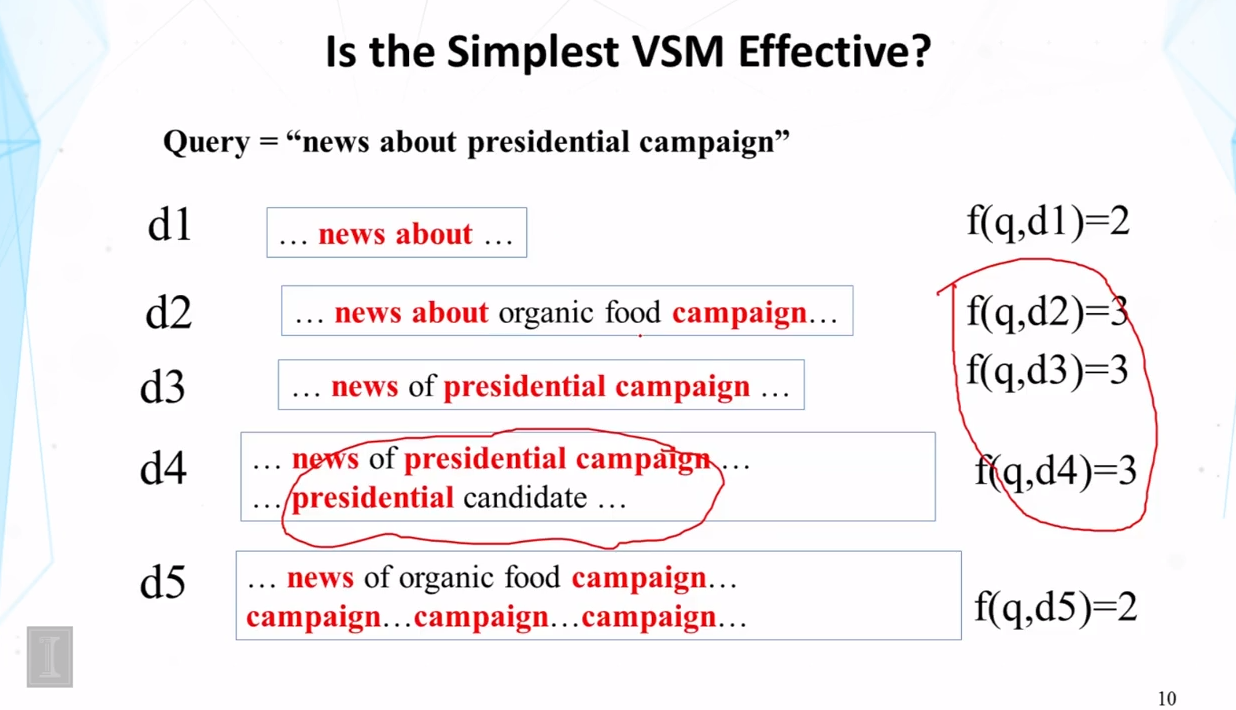1. 匹配了多次“presidential”的文档，应该得到更大的权重

### 2.改进的向量表征：关键词频向量（Improved Vector Placement：Term Frequency Vector）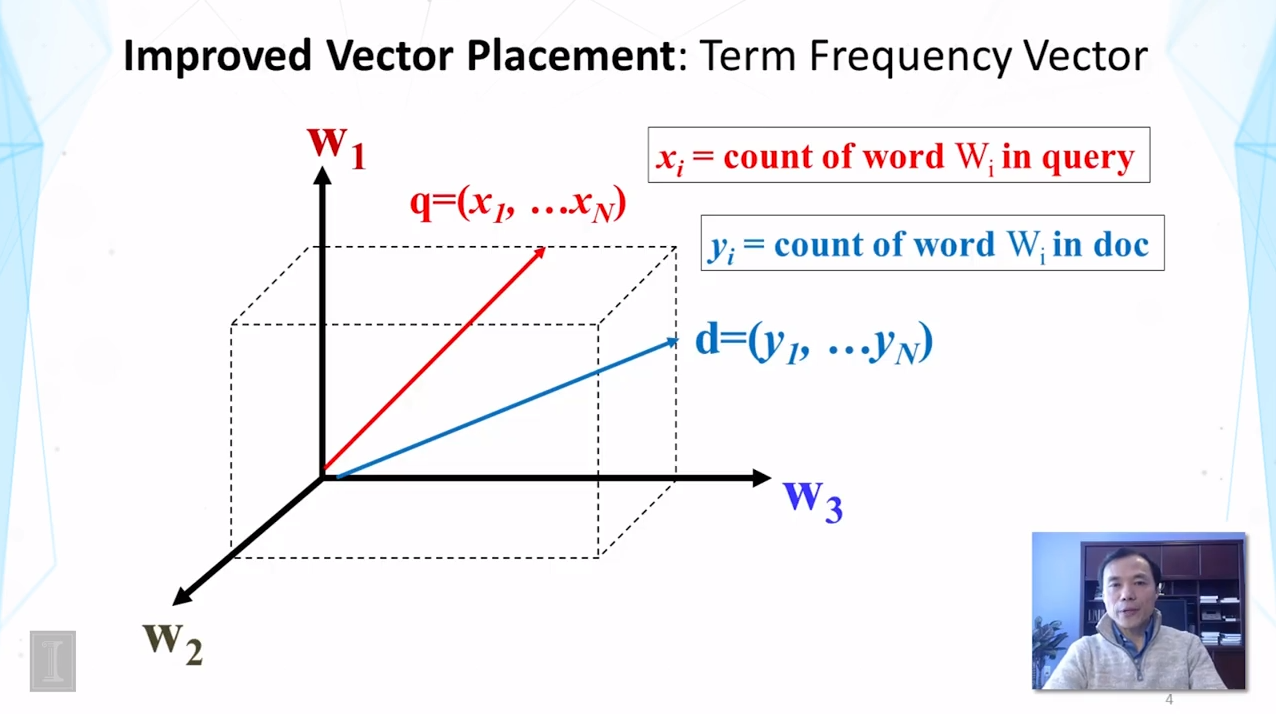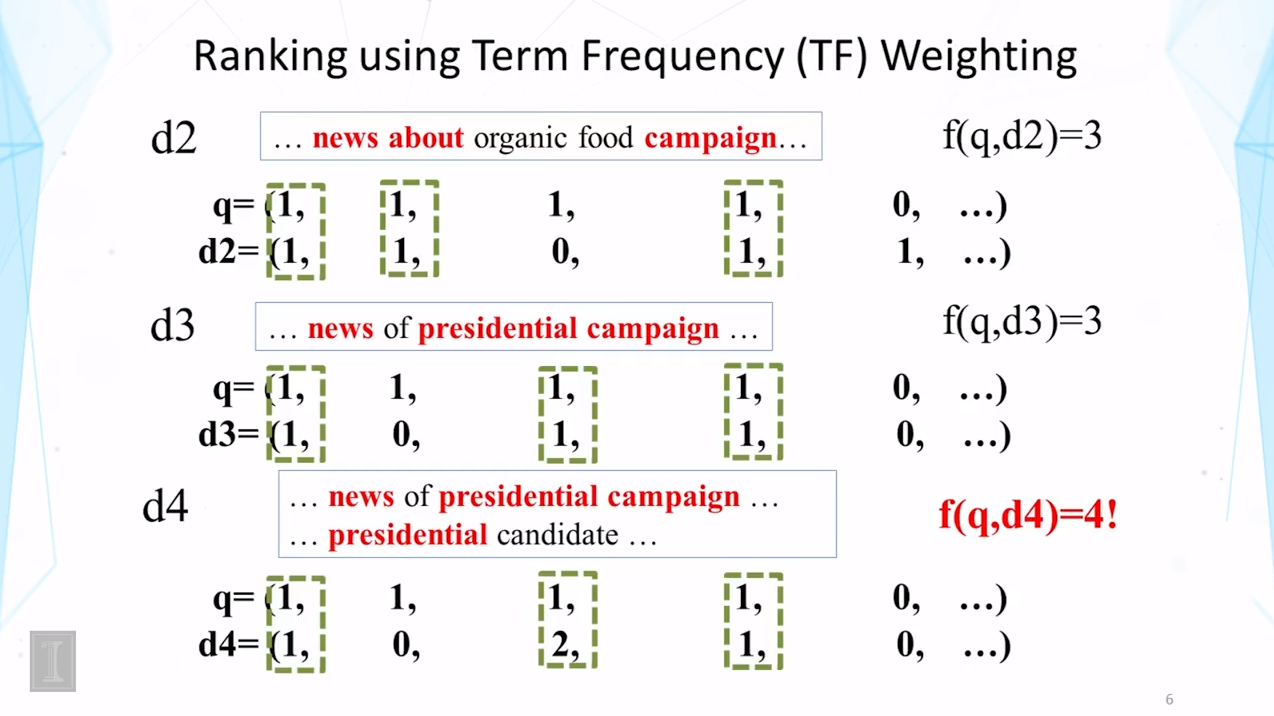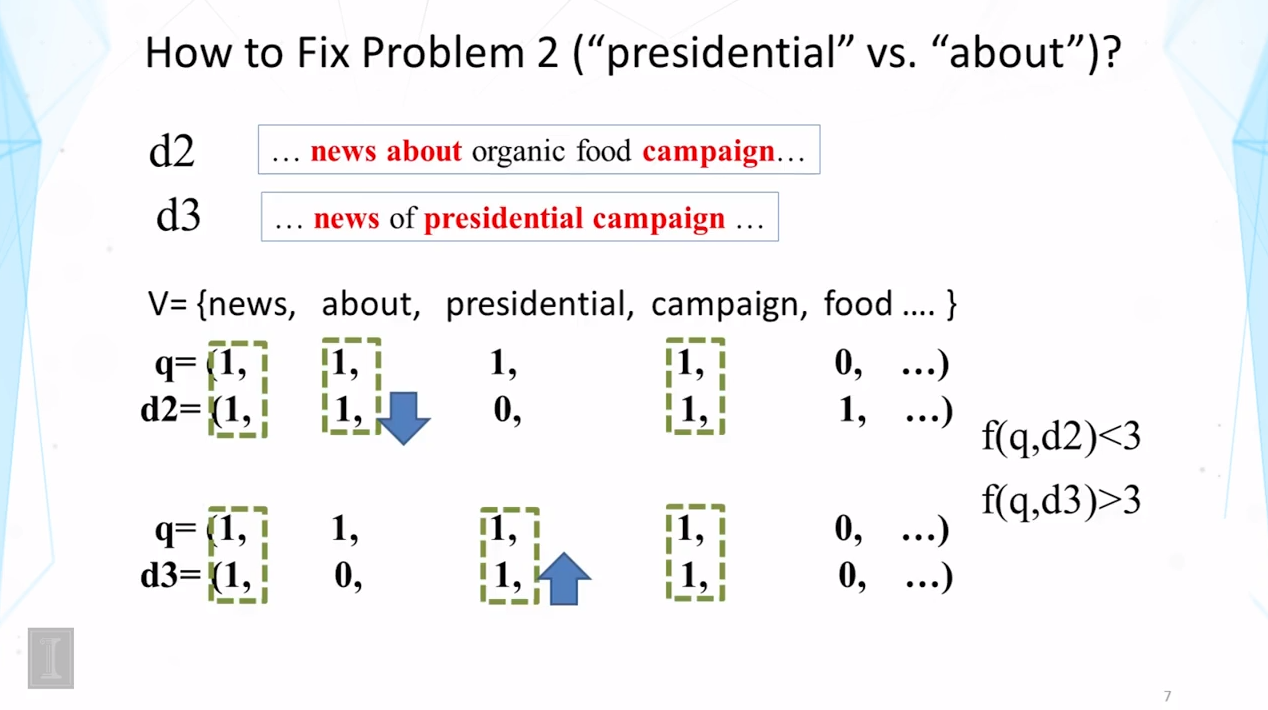### 3.进一步改进的向量表征：转置文档频率（Further Improvement of Vector Placement：Adding Inverse Document Frequency， IDF）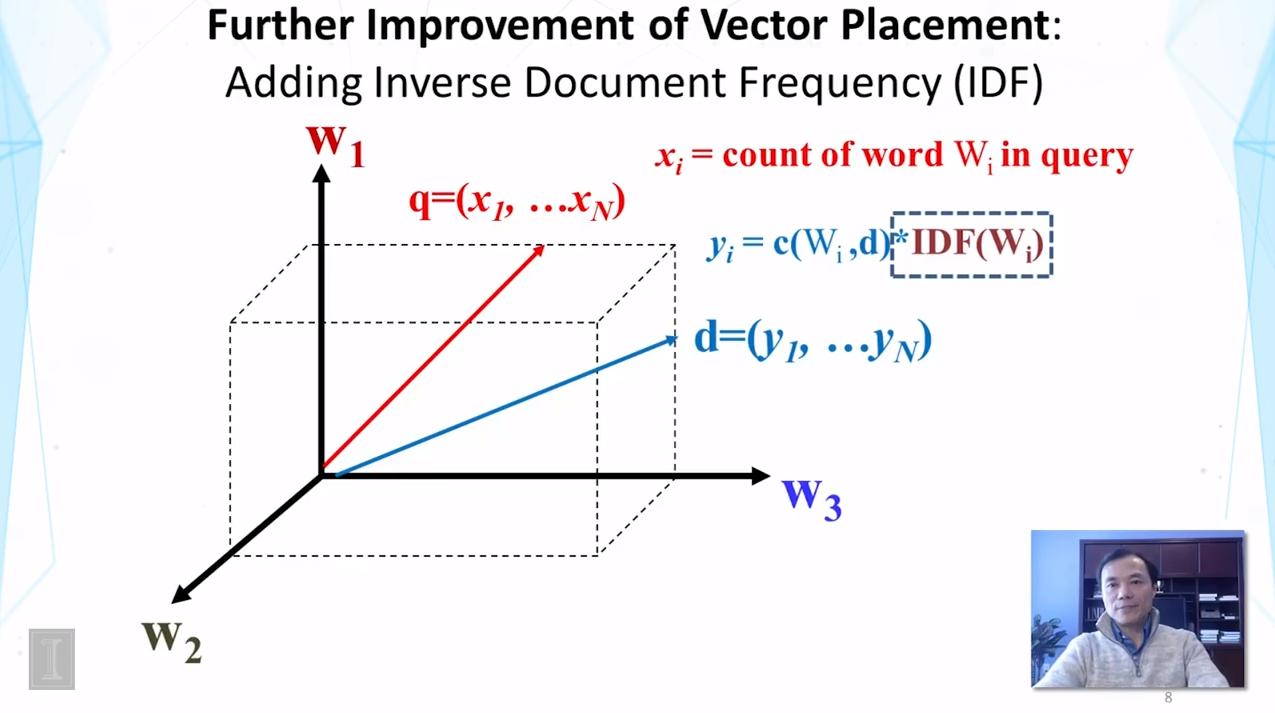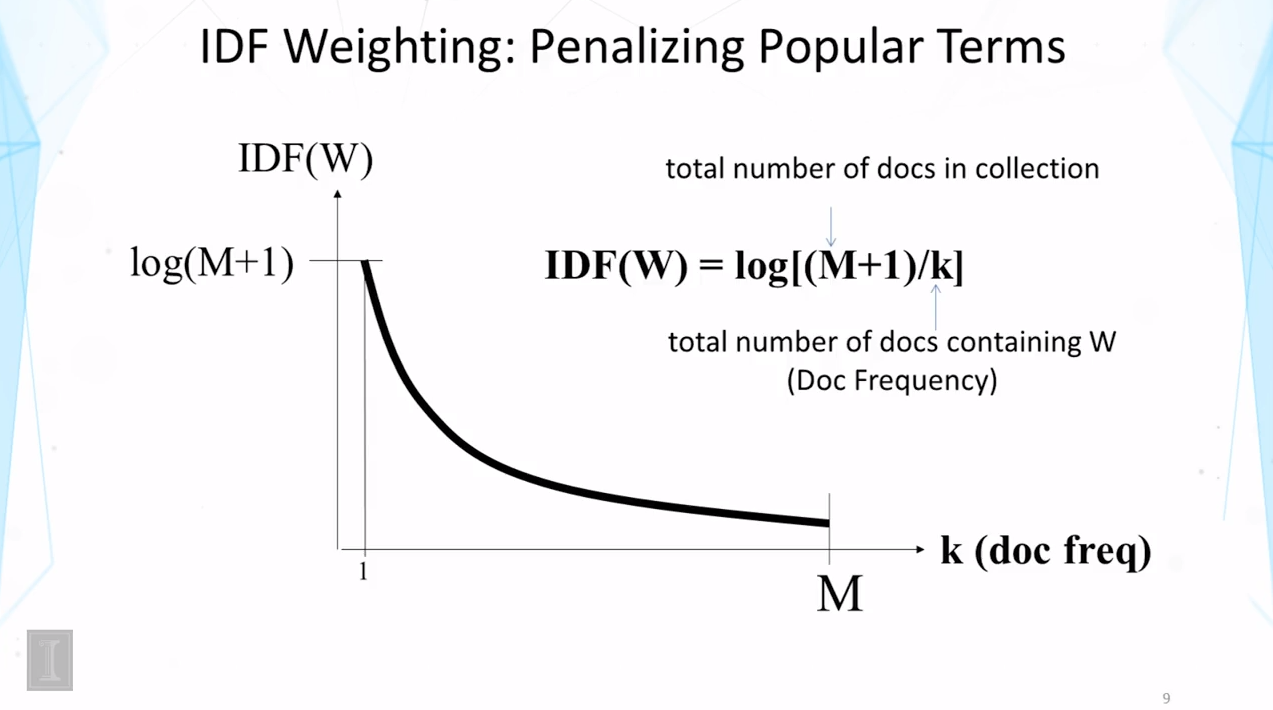$IDF(W)=log[(M+1)/k]$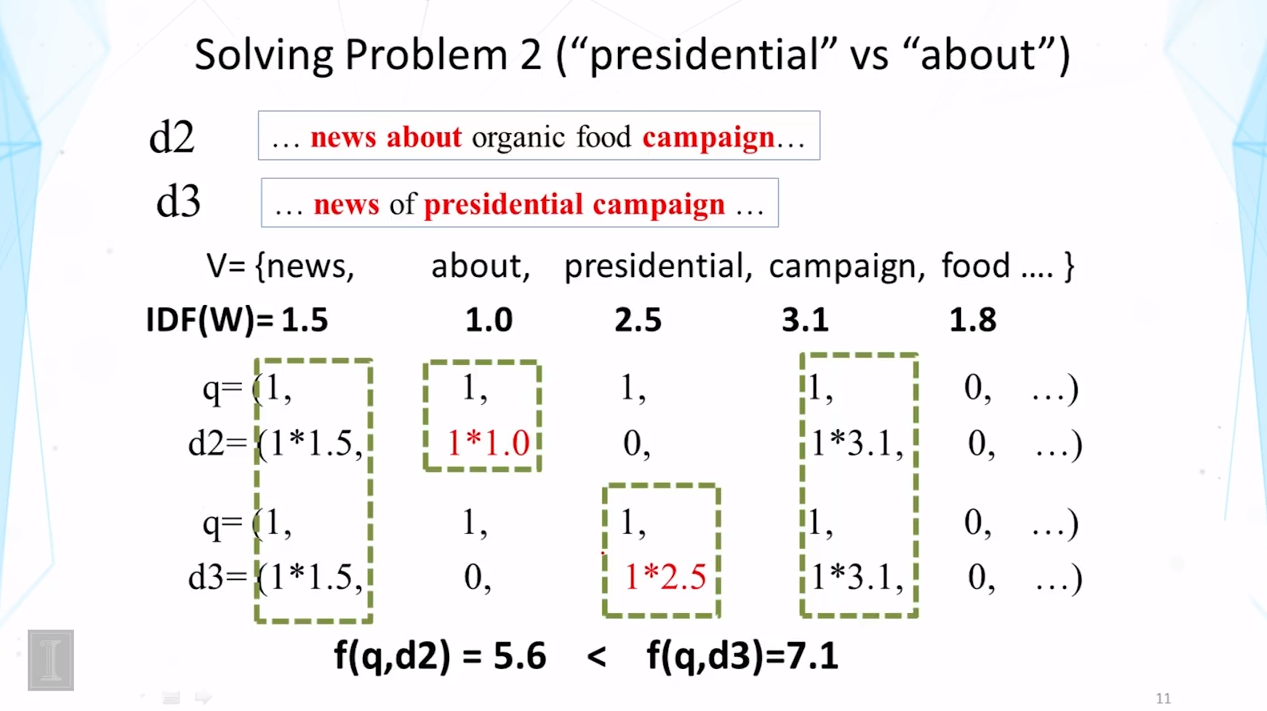### 3.使用了TF-IDF权重算法的VSM模式（How Effective is VSM with TF_IDF Weighting）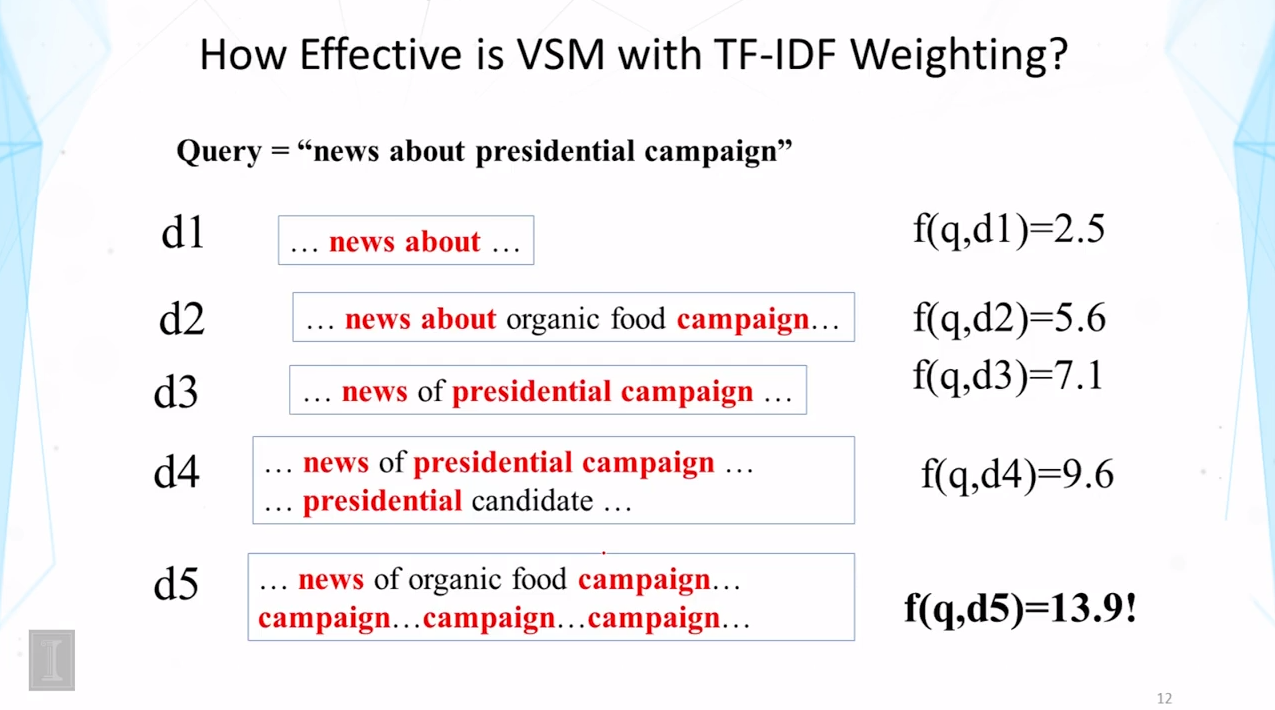## 二、关键词频转换（TF Transformation）

### 1.TF-IDF的实现细节以及存在的问题（Ranking Function with TF-IDF Weighting ）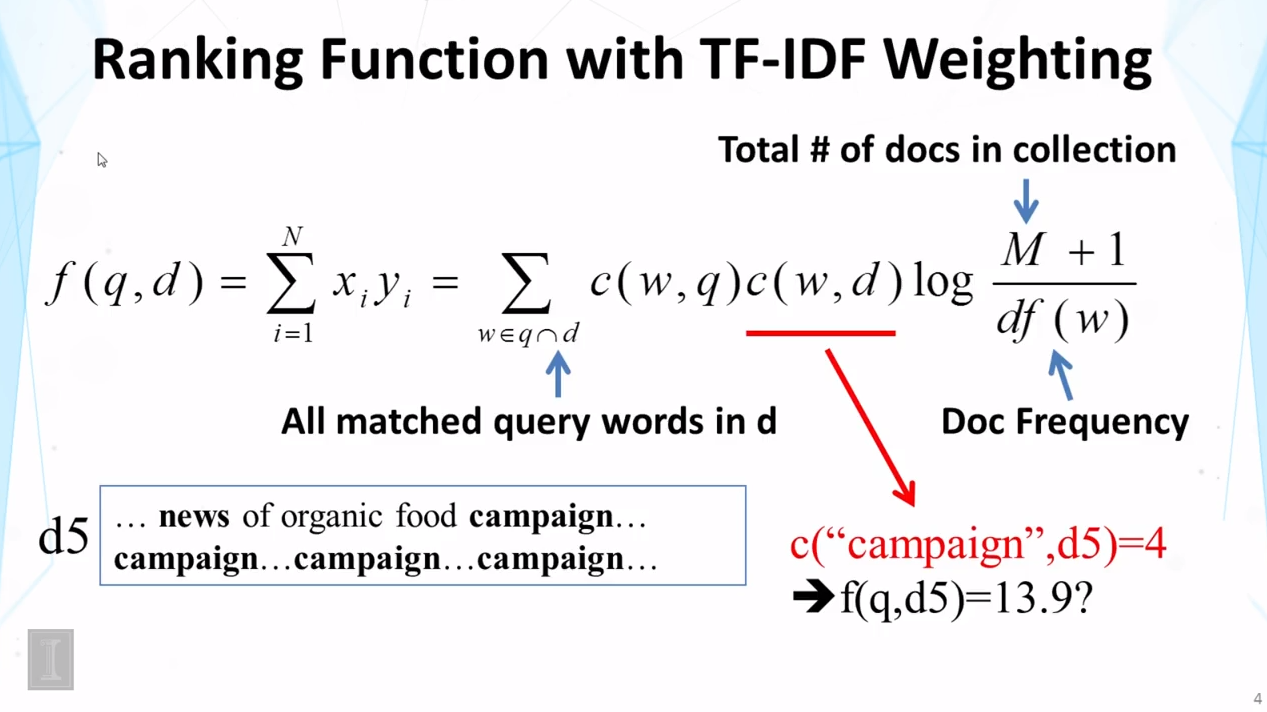（可能有人会问，如果一个关键词反复出现了，那它的IDF是不是降低了，这样能否抵消TF很高带来的影响？答案是否定的，此处的IDF定义的是“包含关键词W的文档数”，只在文档层面统计；而TF表示的是“所有文档中某个关键词出现的总次数”，在文本层面统计了）

### 2.TF转换（TF Transformation）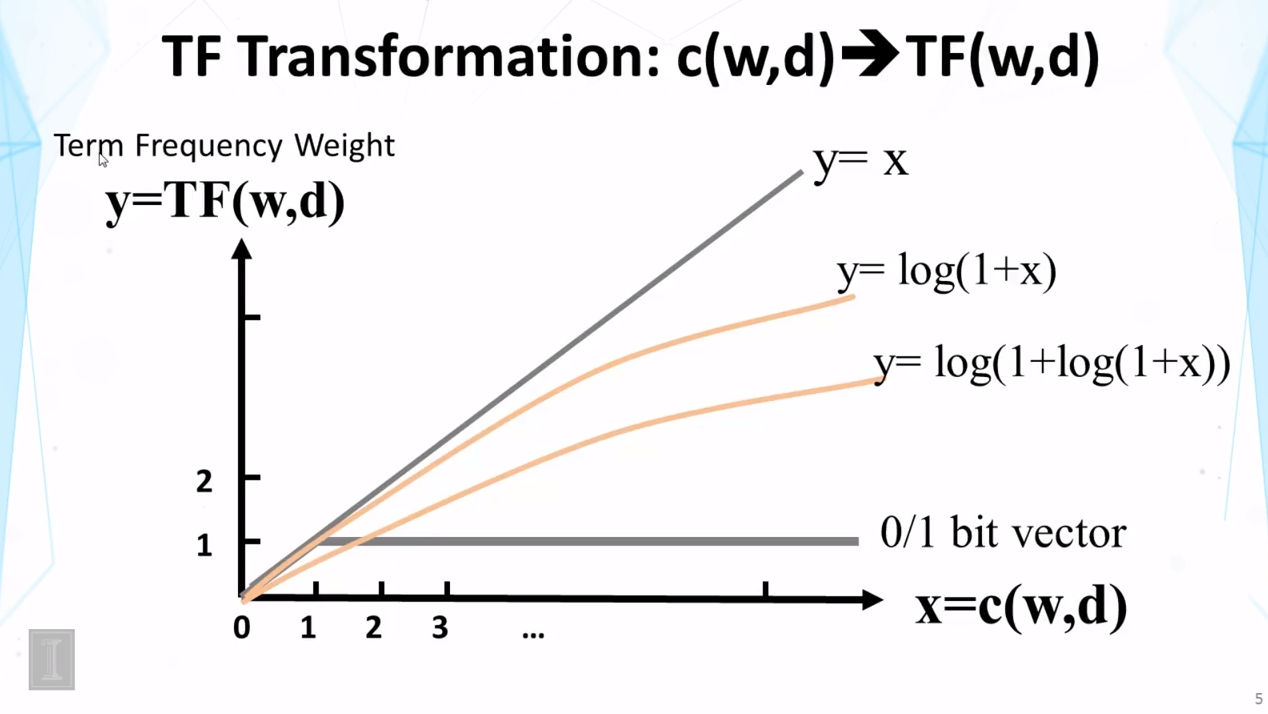$y=\frac{(k+1)x}{x+k}$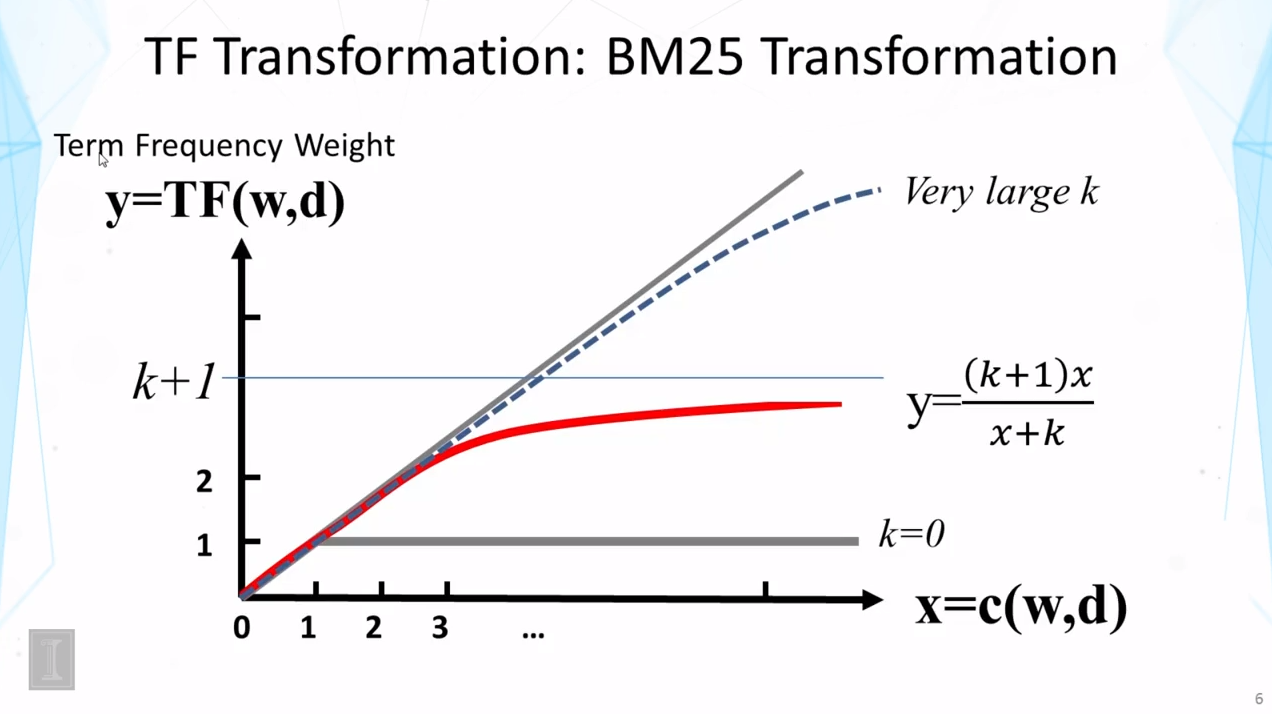### 3.总结

• 次线性TF转换（sublinear TF transformation）可以用来
-对于高关键词频的情况实现“回报抵减”
-避免出现一个关键词支配一切的情况
• BM25转换
-具有上界
-稳定且有效
• 使用BM25 TF的排名函数（k>0）

$f(q,d)=\sum_{i=1}^Nx_iy_i=\sum_{w∈q∩d}c(w,q)\frac{(k+1)c(w,d)}{c(w,d)+k}log\frac{M+1}{df(w)}$

## 三、文档长度标准化（Doc Length Normalization）

### 1.枢轴长度标准化（Pivoted Length Normalization）

• 使用文档长度标准化来惩罚一篇较长的文档
-长文档有更多的机会来匹配任何问题
-需要避免过度惩罚
• 一篇文档较长是因为
-它使用了更多的单词->多一些惩罚
-它拥有更多的内容->少一些惩罚
（这意味着，我们并不总是希望惩罚长文档。一个通俗的例子是，直接将几篇文档拼接为一篇新文档）
• 枢轴长度标准化：使用平均文档长度来作“枢轴”
-如果文档长度=平均长度，则标准化=1

$normalizer=1-b+b\frac{d}{avdl}$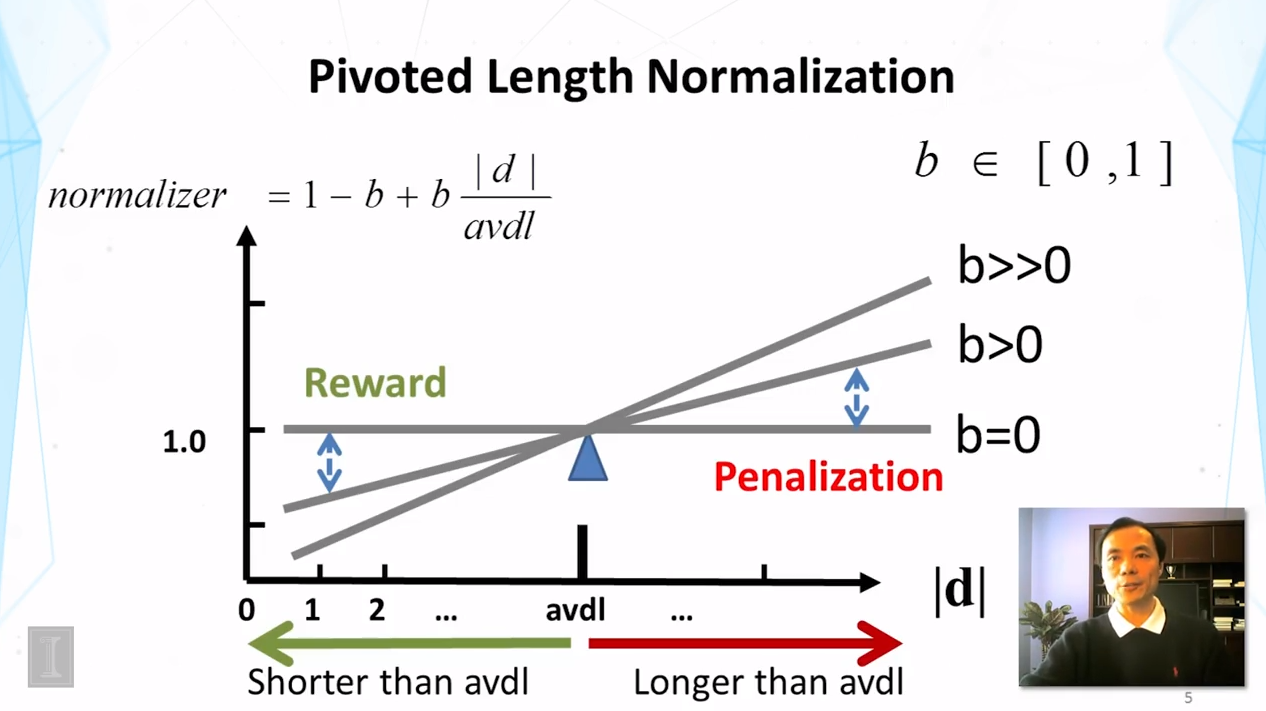### 2.目前最先进的VSM排名函数（State of the Art VSM Ranking Functions）

• 枢轴长度标准化VSM [Singhal et al 96]

$f(q,d)=\sum_{w∈q⌒d}c(w,q)\frac{ln[1+ln[1+c(w,d)]]}{1-b+b\frac{|d|}{avdl}}log\frac{M+1}{df(w)}$

• BM25/Okapi [Robertson & Walker 94]

$f(q,d)=\sum_{w∈q∩d}c(w,q)\frac{(k+1)c(w,q)}{c(w,q)+k(1-b+b\frac{|d|}{avdl})}log\frac{M+1}{df(w)}$

$b∈[0,1] \\ k_1,k_3∈[0,+∞)$

### 3.更进一步优化VSM（Further Improvement of VSM）

• 提升维度的实例化
-词干词，停用词删除，短语，潜在语义指数（单词聚类）
-包含短语的词包通常更有效
-语言和语域标记对于确保“关键词标准化”很重要
• 提升相似函数的实例化
-两向量的余弦函数值
-欧氏距离？
-点积似乎仍然是最好的（在使用了合理的关键词权重时具有良好的泛用性）

### 4.更进一步优化BM25（Further Improvement of BM25）

• BM25F [Robertson & Zaragoza 09]
-使用BM25来处理文档（“F”=fields）
-关键想法：连接所有fields的关键词频，然后使用BM25（而不是其他的）
• BM25+ [Lv & Zhai 11]
-通过向TF添加一个常数，优化原BM25惩罚长文档的部分。
-在理论上和实践上的表现都优于BM25

### 5.对向量空间模式的总结（Summary of Vector Space Model）

• 相关性（q,d）= 相似度（q,d）
• 询问和文档都用向量来表示
• 启发式的排名函数
• 主要关键词的启发
-TF权重和转换
-IDF权重
-文档长度标准化
• BM25和枢轴标准化看起来最有效

## 四、文本检索系统的实施（Implementation of TR Systems）

### 1.典型的TR系统结构（Typical TR System Architecture）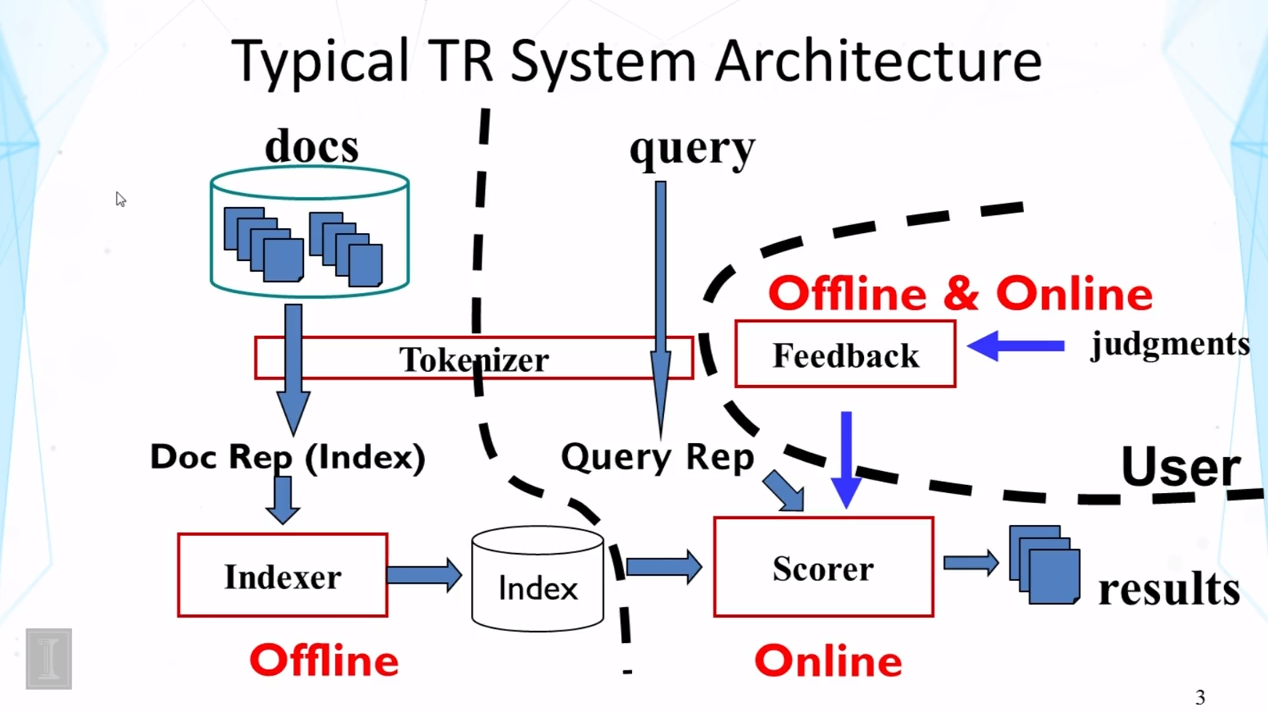### 2.标记化（Tokenization）

• 词汇单位标准化：具有相同意思的单词应该被映射到同一个指数项上面
• 流：将单词的各种形式映射到同一个词根形式，例如
– computer->compute
– computation->compute
– computing->compute
• 一些语言（例如中文）的存储形式会带来挑战

### 3.索引化（Indexing）

• 索引化=将文档转化为支持快速搜索的数据结构（做出更多的预处理）
• 转置索引是用于基本搜索算法领域最流行的索引化方法
• 可能需要其他索引（例如 文档索引）来做反馈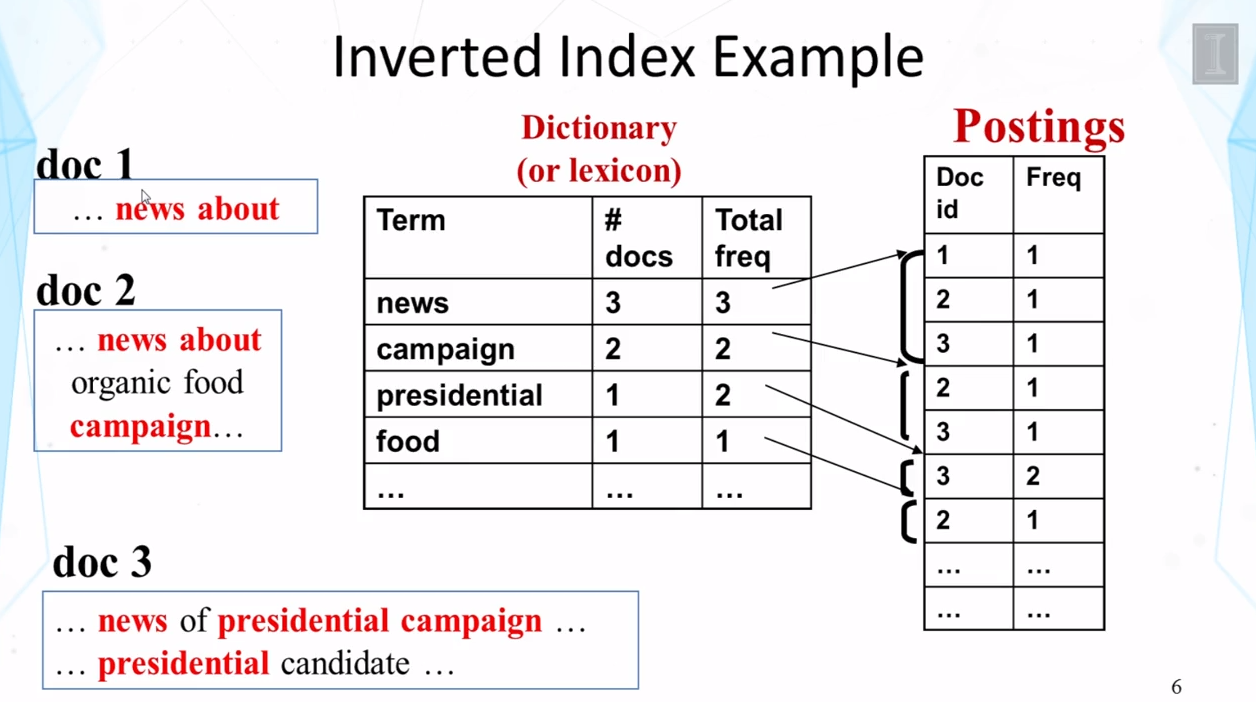### 4.转置索引应用于快速搜索（Inverted Index for Fast Search）

• 单关键词询问？
• 多关键词布尔询问？
-需要匹配 “A” AND “B”
-需要匹配 “A” OR “B”
• 多关键词的核心关键词提问
-与分离的布尔询问相似（”A” OR “B”）
-整合了关键词权重
• 比顺序搜索文档更有效（为什么？）

### 5.单词的经验分布（Empirical distribution of words）

• 有很多与具体语言无关的自然语言处理方法
• 有一些单词出现得非常频繁（例如”the”,”a”,”we”）；但是大多数单词出现得很少。
• 在一篇文献中出现得最多的单词，可能在另一篇中出现得很少

### 6.齐夫定律（Zipf’s Law）

$F(w)=\frac{C}{r(w)^\alpha} \\\alpha≈1,C≈0.1$

### 7.转置索引的数据结构（Data Structures for Inverted Index）

• 词典：尺寸适中
-需要快速的随机访问
-最好是在内存中
-哈希表，B树等等
• 数量：巨大
-希望能顺序访问
-能够存储在硬盘上
-可能会包含文档ID，关键词频，关键词条等等
-需要压缩数据
Tags:##### IIll

index不要翻译成指数，翻译成“索引”比较合适。##### zhouyongyi

$${}$$